# Infinite limits - vertical asymptotes

### Infinite limits - vertical asymptotes

Limits don't always necessarily give numerical solutions. What happens if we take the limit of a function near its vertical asymptotes? We will answer this question in this section, as well as exploring the idea of infinite limits using one-sided limits and two-sided limits.

#### Lessons

i)
${\;}\lim_{x \to {a^ - }} f\left( x \right) =\infty$
ii)
$\lim_{x \to {a^ + }} f\left( x \right) =\infty$
iii)
$\lim_{x \to {a^ - }} f\left( x \right) =,- \infty$
iv)
$\lim_{x \to {a^ + }} f\left( x \right) =,- \infty$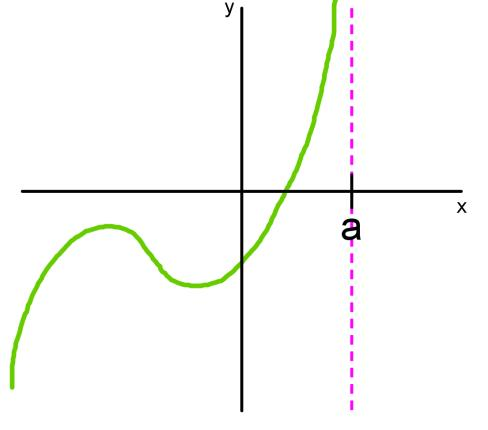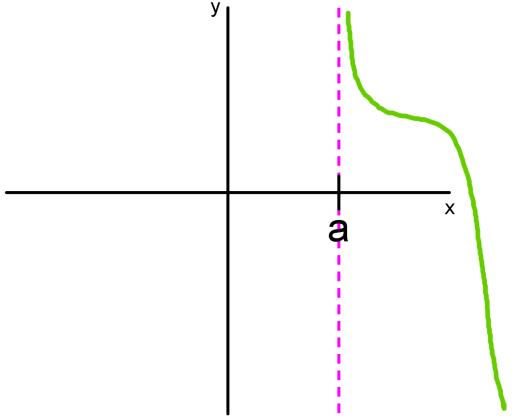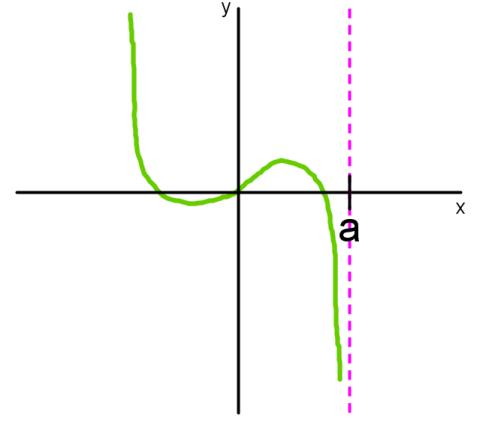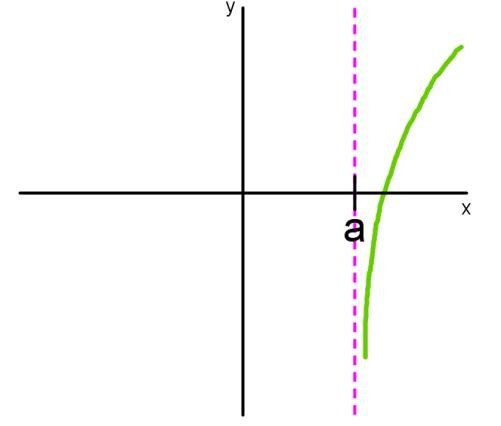• Introduction
Introduction to Vertical Asymptotes
a)
finite limits VS. infinite limits

b)
infinite limits translate to vertical asymptotes on the graph of a function

c)
vertical asymptotes and curve sketching

• 1.
Determine Infinite Limits Graphically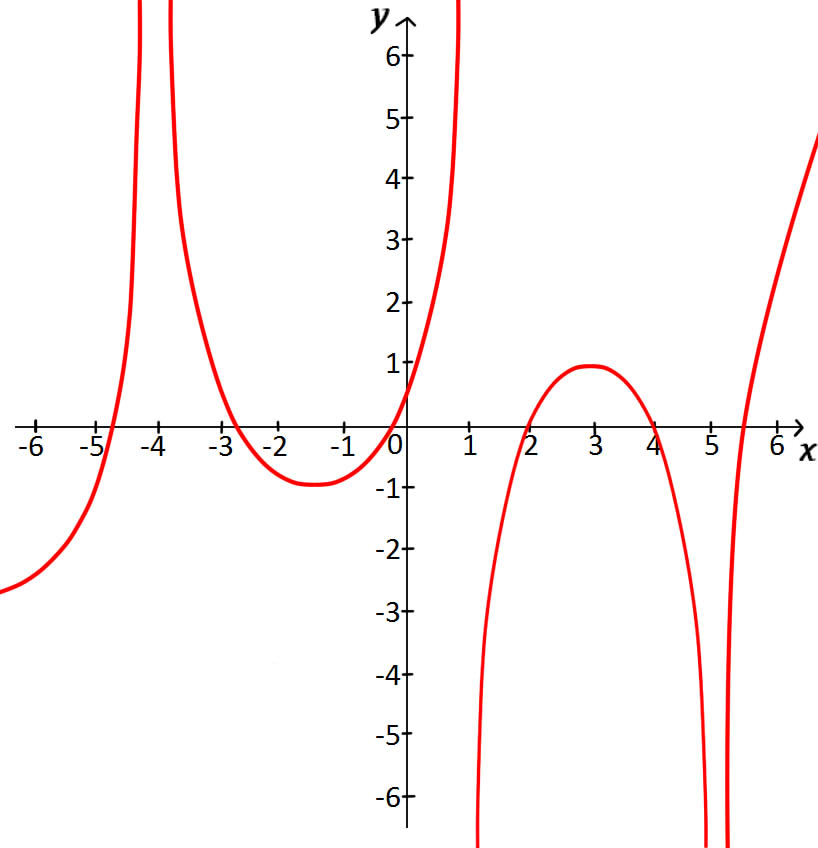For the function $f$ whose graph is shown, state the following:
a)
$\lim_{x \to - {4^ - }} \;f\left( x \right)$
$\lim_{x \to - {4^ + }} \;f\left( x \right)$
$\lim_{x \to - 4} \;f\left( x \right)$

b)
$\lim_{x \to {1^ - }} \;f\left( x \right)$
$\lim_{x \to {1^ + }} \;f\left( x \right)$
$\lim_{x \to 1} \;f\left( x \right)$

c)
$\lim_{x \to {3^ - }} \;f\left( x \right)$
$\lim_{x \to {3^ + }} \;f\left( x \right)$
$\lim_{x \to 3} \;f\left( x \right)$

d)
$\lim_{x \to {5^ - }} \;f\left( x \right)$
$\lim_{x \to {5^ + }} \;f\left( x \right)$
$\lim_{x \to 5} \;f\left( x \right)$

• 2.
Evaluate Infinite Limits Algebraically
Find:
a)
$\lim_{x \to {0^ - }} \;\frac{1}{x}$
$\lim_{x \to {0^ + }} \;\frac{1}{x}$
$\lim_{x \to 0} \;\frac{1}{x}$

b)
$\lim_{x \to {0^ - }} \;\frac{1}{{{x^2}}}$
$\lim_{x \to {0^ + }} \;\frac{1}{{{x^2}}}$
$\lim_{x \to 0} \;\frac{1}{{{x^2}}}$

• 3.
Evaluate Limits Algebraically
Find:
$\lim_{x \to {2^ - }} \;\frac{{5x}}{{x - 2}}$
$\lim_{x \to {2^ + }} \;\frac{{5x}}{{x - 2}}$
$\lim_{x \to 2} \;\frac{{5x}}{{x - 2}}$

• 4.
Determine Infinite Limits of Log Functions
Determine:
$\lim_{x \to {0^ + }} \ln x$